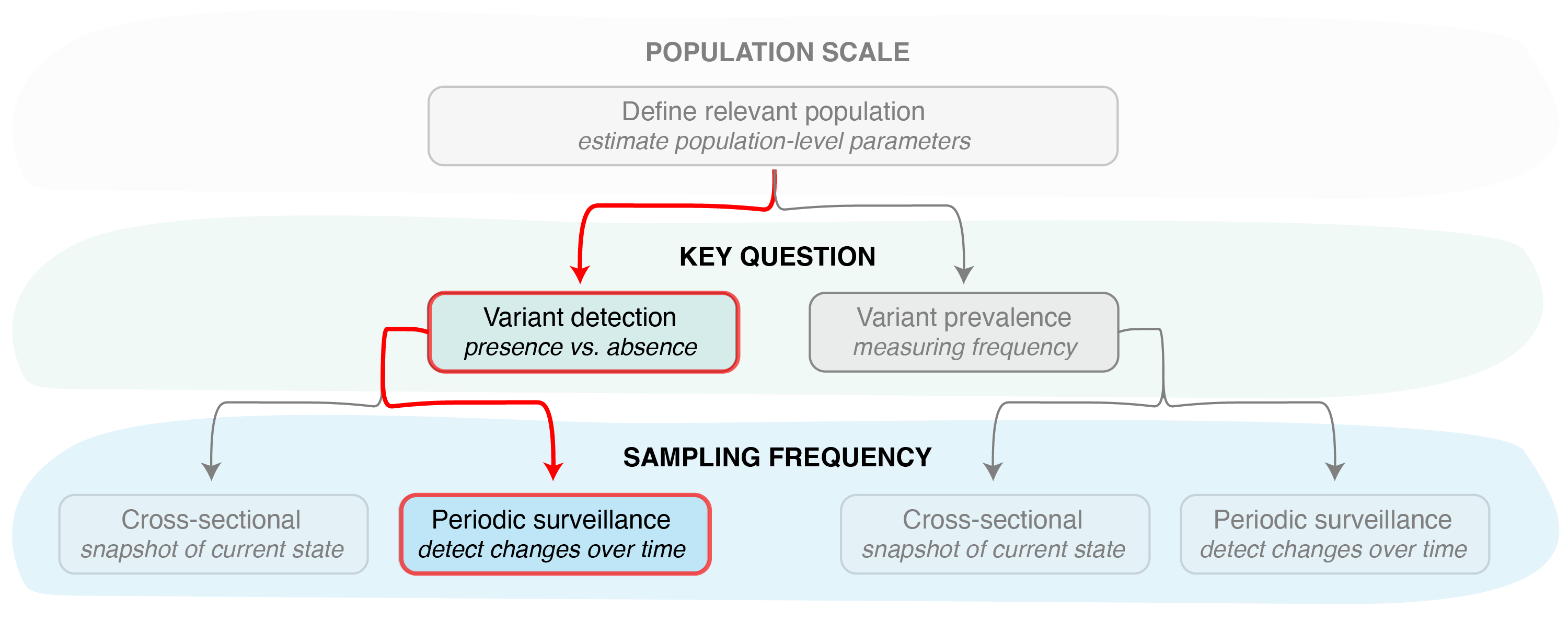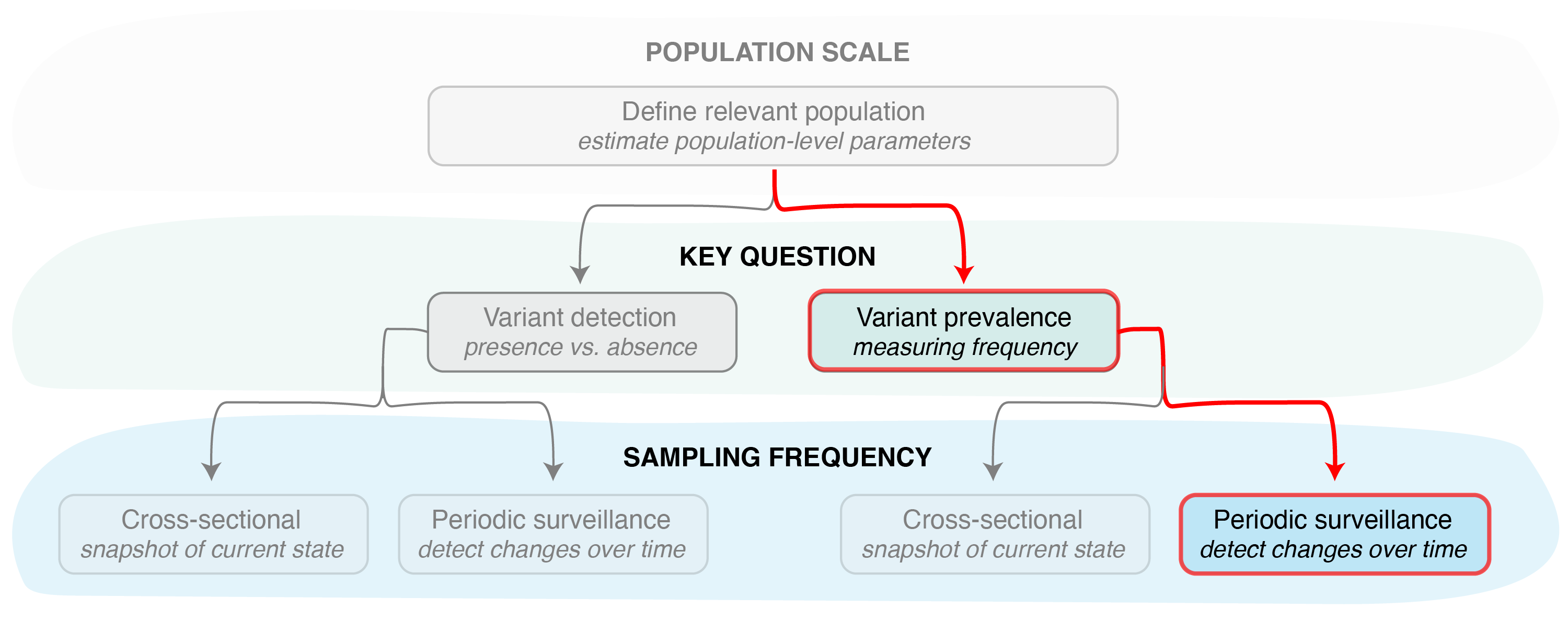# Estimating the probability of detecting a variant

### Periodic sampling

This vignette provides an overview of the functions that can be used to estimate the probability of detecting a pathogen variant in a population, given a periodic sampling scheme with a fixed sample size.Figure 1. Identifying the key question (detection vs prevalence) is the second step in designing a pathogen surveillance system. Determining confidence in the result (e.g., the probability of detection or confidence in frequency estimation) then requires determining the sampling frequency (cross-sectional or periodic).

By specifying sampling_freq = "cont", the vartrack_samplesize_detect() function can be used to calculate the probability of detecting a particular variant in the population within a specific number of days since its introduction, OR by the time the variant reaches a specific frequency. As with the cross-sectional option (sampling_freq = "xsect"; see Estimating the probability of detecting a variant: cross-sectional), this requires knowledge of the coefficient of detection ratio between two pathogen variants (or, more commonly, one variant and the rest of the pathogen population). The coefficient of detection ratio for two variants can be calculated using the vartrack_cod_ratio() function (see Estimating bias in observed variant prevalence for more details). Since we are only interested in the ratio of the coefficients of detection, applying this function only requires providing parameters which are expected to differ between variants. The ratio between any variants not provided is assumed to be equal to one.

Once we have an estimate of the coefficient of detection ratio, we can calculate the sample size needed for detection from the following parameters:

Param Variable Name Description
$$n$$ n the per-day sample size
$$t$$ t the number of days after introduction a variant should be detected by
$$P_{V_1}$$ p_detect the desired prevalence to detect a variant by
$$\omega$$ omega the sequencing success rate
$$P_{0_{V_1}}$$ p0_v1 the initial variant prevalence (# introductions / population size)
$$r_{V_1}$$ r_v1 the estimated logistic growth rate of the variant (per day)
$$\frac{C_{V_1}}{C_{V_2}}$$ c_ratio the coefficient of detection ratio, calculated as the ratio of the coefficients of variant 1 to variant 2 (can be calculated using vartrack_cod_ratio())

To calculate the probability of detection assuming periodic sampling, we must provide either the number of days after introduction a variant should be detected by ($$t$$) OR the desired prevalence to detect a variant by ($$P_{V_1}$$), but not both. All other parameters listed above are required.

Therefore, if we would like to know the probability of detecting a variant by the time it reaches 1% prevalence in the population, we can apply the vartrack_prob_detect() function as follows:

library(phylosamp)
c1_c2 <- vartrack_cod_ratio(phi_v1=0.975, phi_v2=0.95, gamma_v1=0.8, gamma_v2=0.6)
vartrack_prob_detect(n=20, p_v1=0.01, omega=0.8, p0_v1=3/10000,
r_v1=0.1, c_ratio=c1_c2, sampling_freq="cont")
## Calculating probability of detection assuming periodic sampling
##  0.9018312

In other words, we have a 90% probability of detecting a variant by the time it reaches a frequency of 1% in the population if we sequence 20 samples per day (which results in 16 high-quality sequences that can be used for characterization, due to $$\omega=0.8$$).

If instead we are interested in detecting a variant within the first month of its introduction into the population (assuming all other parameters are the same as above), we can use the calc_prob_detect() function as follows:

c1_c2 <- vartrack_cod_ratio(phi_v1=0.975, phi_v2=0.95, gamma_v1=0.8, gamma_v2=0.6)
vartrack_prob_detect(n=20, t=30, omega=0.8, p0_v1=3/10000, r_v1=0.1,
c_ratio=c1_c2, sampling_freq="cont")
## Calculating probability of detection assuming periodic sampling
##  0.7130925

Here we can see that we have only a 71% chance of detecting the variant within 30 days given the same sampling scheme and sequencing success rate.

##### Variant prevalenceFigure 2. The phylosamp package currently does not provide functionality for estimating the confidence in a prevalence estimate given a periodic sampling strategy.

The phylosamp package currently does not provide functionality for estimating the confidence in a prevalence estimate given a periodic sampling strategy. However, it can be used to calculate the sample size needed given a confidence level and either periodic or cross-sectional sampling strategies.